# Debugging with Visual Studio .NET

In the next sections, you will learn how to debug the sample application. The sample application contains several syntax errors and a few "features" that the client did not expect. By stepping through and debugging a sample application, you will become familiar with the purpose of the debugger, and learn how to use it to be more productive by creating more stable, reliable, and efficient code.

#### Debugging an Application

When trying to fix syntax errors, it is not necessary to know what the application is attempting to accomplish. However, when attempting to debug an application, it is helpful, and in most cases a necessity, to know what the application is supposed to do. The following application, Listing 42.1, is a simple program that calculates the average scores in a class. It also prints out the smartest and least smartest person in the class (based upon grades).

##### Listing 42.1. Sample Program with Syntax Errors
` using System; using System.Collections; namespace SAMS.VisualCSharpUnleashed.Chapter45 {   class Student   {     private string  m_name;     private double  m_grade;     public Student(string name, double grade)     {       m_name  = name;       m_grade = grade;     }     public double Grade     {       get { return m_grade; }       set { m_grade = value; }     }     public string Name     {       get { return m_name; }       set { m_name = value; }     }   }   class SimpleDebuggingClass   {     private System.Collections.ArrayList m_list = new System.Collections.ArrayList();     protected void CreateStudentList()     {       m_list.Add(new Student("Lonny",   100.0));       m_list.Add(new Student("Richard", 90.0));       m_list.Add(new Student("Ahmed",   92.5));       m_list.Add(new Student("Jeff",    75.0));       m_list.Add(new Student("Neal",    46.8));       m_list.Add(new Student("Roman",   70.0));     }     protected double CalculateAverageGrade()     {       double totalScores = 0;       for(int i=0; i<m_list.Count; i++)       {         totalScores += ((Student) m_list[i]).Grade;       }       return totalScores / m_list.Count;     }     protected Student GetSmartest()     {       double highestScore = 0;       Student result      = null;       for(int i=0; i<m_list.Count; i++)       {         if(highestScore < ((Student) m_list[i]).Grade)         {           highestScore = ((Student) m_list[i]).Grade;           result = (Student) m_list[i];         }       }       return result;     }     protected Student GetLeastSmartest()     {       double lowestScore  = 0;       Student result      = null;       for(int i=0; i<m_list.Count(); i++)       {         if(lowestScore > ((Student) m_list[i]).Grade)         {           lowestScore = ((Student) m_list[i]).Grade;           result = (Student) m_list[i];         }       }       return result;     }     [STAThread]     static void Main(string[] args)     {       SimpleDebuggingClass sample = new SimpleDebuggingClass();       sample.CreateStudentList();       // Calculate and print the average grade in the class.       double avgGrade = sample.CalculateAverageGrade()       System.Console.WriteLine("Average grade in the class is: {0}", avgGrade);       // Calculate the smartest and "least smartest" student in the class.       Student smartest      = sample.GetSmartest();       Student leastSmartest = sample.GetLeastSmartest();       System.Console.WriteLine("The smartest person in the class is: {0}", smartest.Name);       System.Console.WriteLine("The least smartest person in the class is:         {0}", leastSmartest.Name);       System.Console.ReadLine();     }   } } `

When you compile this program you will see, as shown in Figure 42.24, that it generates two syntax errors.

##### Figure 42.24. Two syntax errors generated from the sample program.If you double-click on the first error, Visual Studio .NET will take you to the offending line of code, as in Figure 42.25. You will notice that this error states that 'System.Collections.ArrayList.Count' denotes a 'property' where a 'method' was expected. This simply means that a method was made out of a property. Remove the () from the line:

` for(int i=0; i<m_list.Count(); i++) `

##### Figure 42.25. First syntax error generated from the sample program.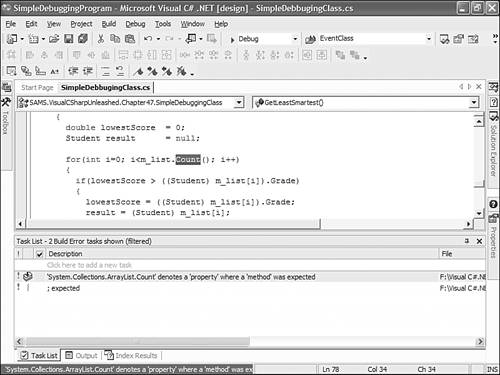If you double-click on the second error, you will notice that this statement is just missing the semicolon (;) character, as in Figure 42.26. Simply add the semicolon to the end of this line and recompile.

##### Figure 42.26. Second syntax error generated from the sample program.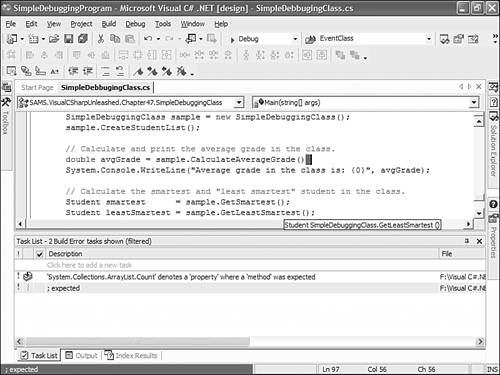The application should now compile. However, if you run the application, you will notice a more menacing problem. Running the application cause an exception to be thrown, as in Figure 42.27.

##### Figure 42.27. Exception thrown from the application.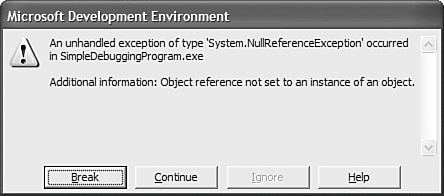Fortunately, if you click the Break button in this message, the debugger places you at the offending line. If you put your cursor over the leastSmartest variable, you will notice that it is set to null, as shown in Figure 42.28.

##### Figure 42.28. The offending line of code.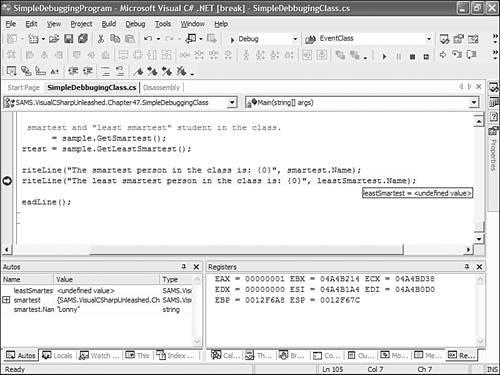Now that you know that this is the problem causing the exception, you can start looking at the code where this variable is being set. However, because this is a simple application, there is no way to re-execute the code. When you restart the code, you will want to stop before the variable is set. This will enable you to inspect the process as it is happening. To cause the application to stop, set a breakpoint at the following line:

` Student leastSmartest = sample.GetLeastSmartest(); `

Now run the application. When the debugger reaches this line, it will stop. Next, step into the method call and make the Watch window visible. Add the following variables to the Watch window:

` (Student) m_list[i] lowestScore `

Now step through the loop and inspect the variables, as in Figure 42.29.

##### Figure 42.29. Inspecting the GetLeastSmartest() method.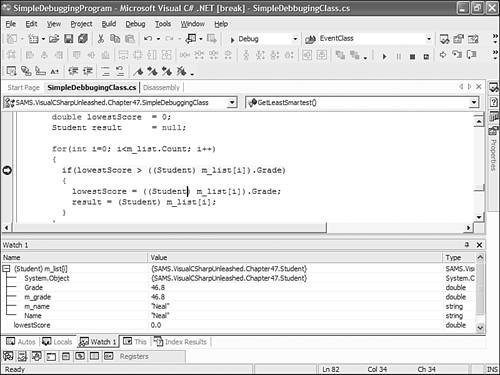Now that you can see the two variables in the Watch window, you will notice that the lowestScore is set to 0.0. Because this was set during initialization, no Student will have a lower grade then this. This means that the leastSmartest Student is always going to be set to null. Set the lowestScore to 100.0 in the initialization and rerun the application. The application now runs without problems. Listing 42.2 contains the corrected version of this application.

##### Listing 42.2. Corrected Sample Program
` using System; using System.Collections; namespace SAMS.VisualCSharpUnleashed.Chapter45 {   class Student   {     private string  m_name;     private double  m_grade;     public Student(string name, double grade)     {       m_name  = name;       m_grade = grade;     }     public double Grade     {       get { return m_grade; }       set { m_grade = value; }     }     public string Name     {       get { return m_name; }       set { m_name = value; }     }   }   class SimpleDebuggingClass   {     private System.Collections.ArrayList m_list = new System.Collections.ArrayList();     protected void CreateStudentList()     {       m_list.Add(new Student("Lonny",   100.0));       m_list.Add(new Student("Richard", 90.0));       m_list.Add(new Student("Ahmed",   92.5));       m_list.Add(new Student("Jeff",    75.0));       m_list.Add(new Student("Neal",    46.8));       m_list.Add(new Student("Roman",   70.0));     }     protected double CalculateAverageGrade()     {       double totalScores = 0;       for(int i=0; i<m_list.Count; i++)       {         totalScores += ((Student) m_list[i]).Grade;       }       return totalScores / m_list.Count;     }     protected Student GetSmartest()     {       double highestScore = 0;       Student result      = null;       for(int i=0; i<m_list.Count; i++)       {         if(highestScore < ((Student) m_list[i]).Grade)         {           highestScore = ((Student) m_list[i]).Grade;           result = (Student) m_list[i];         }       }       return result;     }     protected Student GetLeastSmartest()     {       double lowestScore  = 100.0;       Student result      = null;       for(int i=0; i<m_list.Count; i++)       {         if(lowestScore > ((Student) m_list[i]).Grade)         {           lowestScore = ((Student) m_list[i]).Grade;           result = (Student) m_list[i];         }       }       return result;     }     [STAThread]     static void Main(string[] args)     {       SimpleDebuggingClass sample = new SimpleDebuggingClass();       sample.CreateStudentList();       // Calculate and print the average grade in the class.       double avgGrade = sample.CalculateAverageGrade();       System.Console.WriteLine("Average grade in the class is: {0}", avgGrade);       // Calculate the smartest and "least smartest" student in the class.       Student smartest      = sample.GetSmartest();       Student leastSmartest = sample.GetLeastSmartest();       System.Console.WriteLine("The smartest person in the class is: {0}",         smartest.Name);       System.Console.WriteLine("The least smartest person in the class is: {0}",         leastSmartest.Name);       System.Console.ReadLine();     }   } } `Visual C#. NET 2003 Unleashed
ISBN: 672326760
EAN: N/A
Year: 2003
Pages: 316